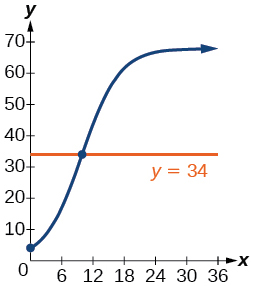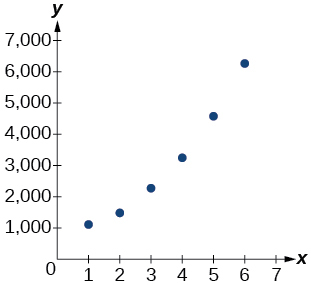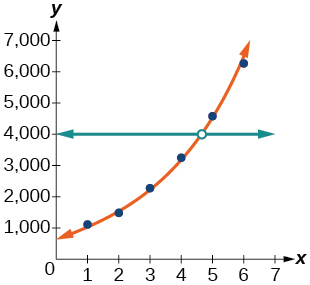# 6.8 Fitting exponential models to data  (Page 7/12)

 Page 7 / 12

What might a scatterplot of data points look like if it were best described by a logarithmic model?

What does the y -intercept on the graph of a logistic equation correspond to for a population modeled by that equation?

The y -intercept on the graph of a logistic equation corresponds to the initial population for the population model.

## Graphical

For the following exercises, match the given function of best fit with the appropriate scatterplot in [link] through [link] . Answer using the letter beneath the matching graph.

$y=10.209{e}^{-0.294x}$

$y=5.598-1.912\mathrm{ln}\left(x\right)$

C

$y=2.104{\left(1.479\right)}^{x}$

$y=4.607+2.733\mathrm{ln}\left(x\right)$

B

$y=\frac{14.005}{1+2.79{e}^{-0.812x}}$

## Numeric

To the nearest whole number, what is the initial value of a population modeled by the logistic equation $\text{\hspace{0.17em}}P\left(t\right)=\frac{175}{1+6.995{e}^{-0.68t}}?\text{\hspace{0.17em}}$ What is the carrying capacity?

$P\left(0\right)=22\text{\hspace{0.17em}}$ ; 175

Rewrite the exponential model $\text{\hspace{0.17em}}A\left(t\right)=1550{\left(1.085\right)}^{x}\text{\hspace{0.17em}}$ as an equivalent model with base $\text{\hspace{0.17em}}e.\text{\hspace{0.17em}}$ Express the exponent to four significant digits.

A logarithmic model is given by the equation $\text{\hspace{0.17em}}h\left(p\right)=67.682-5.792\mathrm{ln}\left(p\right).\text{\hspace{0.17em}}$ To the nearest hundredth, for what value of $\text{\hspace{0.17em}}p\text{\hspace{0.17em}}$ does $\text{\hspace{0.17em}}h\left(p\right)=62?$

$p\approx 2.67$

A logistic model is given by the equation $\text{\hspace{0.17em}}P\left(t\right)=\frac{90}{1+5{e}^{-0.42t}}.\text{\hspace{0.17em}}$ To the nearest hundredth, for what value of t does $\text{\hspace{0.17em}}P\left(t\right)=45?$

What is the y -intercept on the graph of the logistic model given in the previous exercise?

y -intercept: $\text{\hspace{0.17em}}\left(0,15\right)$

## Technology

For the following exercises, use this scenario: The population $\text{\hspace{0.17em}}P\text{\hspace{0.17em}}$ of a koi pond over $\text{\hspace{0.17em}}x\text{\hspace{0.17em}}$ months is modeled by the function $\text{\hspace{0.17em}}P\left(x\right)=\frac{68}{1+16{e}^{-0.28x}}.$

Graph the population model to show the population over a span of $\text{\hspace{0.17em}}3\text{\hspace{0.17em}}$ years.

What was the initial population of koi?

$4\text{\hspace{0.17em}}$ koi

How many koi will the pond have after one and a half years?

How many months will it take before there are $\text{\hspace{0.17em}}20\text{\hspace{0.17em}}$ koi in the pond?

about $\text{\hspace{0.17em}}6.8\text{\hspace{0.17em}}$ months.

Use the intersect feature to approximate the number of months it will take before the population of the pond reaches half its carrying capacity.For the following exercises, use this scenario: The population $\text{\hspace{0.17em}}P\text{\hspace{0.17em}}$ of an endangered species habitat for wolves is modeled by the function $\text{\hspace{0.17em}}P\left(x\right)=\frac{558}{1+54.8{e}^{-0.462x}},$ where $\text{\hspace{0.17em}}x\text{\hspace{0.17em}}$ is given in years.

Graph the population model to show the population over a span of $\text{\hspace{0.17em}}10\text{\hspace{0.17em}}$ years.

What was the initial population of wolves transported to the habitat?

$10\text{\hspace{0.17em}}$ wolves

How many wolves will the habitat have after $\text{\hspace{0.17em}}3\text{\hspace{0.17em}}$ years?

How many years will it take before there are $\text{\hspace{0.17em}}100\text{\hspace{0.17em}}$ wolves in the habitat?

about 5.4 years.

Use the intersect feature to approximate the number of years it will take before the population of the habitat reaches half its carrying capacity.

For the following exercises, refer to [link] .

 x f(x) 1 1125 2 1495 3 2310 4 3294 5 4650 6 6361

Use a graphing calculator to create a scatter diagram of the data.Use the regression feature to find an exponential function that best fits the data in the table.

Write the exponential function as an exponential equation with base $\text{\hspace{0.17em}}e.$

$f\left(x\right)=776.682{e}^{0.3549x}$

Graph the exponential equation on the scatter diagram.

Use the intersect feature to find the value of $\text{\hspace{0.17em}}x\text{\hspace{0.17em}}$ for which $\text{\hspace{0.17em}}f\left(x\right)=4000.$

When $\text{\hspace{0.17em}}f\left(x\right)=4000,$ $x\approx 4.6.$For the following exercises, refer to [link] .

 x f(x) 1 555 2 383 3 307 4 210 5 158 6 122

Use a graphing calculator to create a scatter diagram of the data.

#### Questions & Answers

The sequence is {1,-1,1-1.....} has
amit Reply
circular region of radious
Kainat Reply
how can we solve this problem
Joel Reply
Sin(A+B) = sinBcosA+cosBsinA
Eseka Reply
Prove it
Eseka
Please prove it
Eseka
hi
Joel
June needs 45 gallons of punch. 2 different coolers. Bigger cooler is 5 times as large as smaller cooler. How many gallons in each cooler?
Arleathia Reply
find the sum of 28th term of the AP 3+10+17+---------
Prince Reply
I think you should say "28 terms" instead of "28th term"
Vedant
if sequence sn is a such that sn>0 for all n and lim sn=0than prove that lim (s1 s2............ sn) ke hole power n =n
SANDESH Reply
write down the polynomial function with root 1/3,2,-3 with solution
Gift Reply
if A and B are subspaces of V prove that (A+B)/B=A/(A-B)
Pream Reply
write down the value of each of the following in surd form a)cos(-65°) b)sin(-180°)c)tan(225°)d)tan(135°)
Oroke Reply
Prove that (sinA/1-cosA - 1-cosA/sinA) (cosA/1-sinA - 1-sinA/cosA) = 4
kiruba Reply
what is the answer to dividing negative index
Morosi Reply
In a triangle ABC prove that. (b+c)cosA+(c+a)cosB+(a+b)cisC=a+b+c.
Shivam Reply
give me the waec 2019 questions
Aaron Reply
the polar co-ordinate of the point (-1, -1)
Sumit Reply

### Read also:

#### Get the best Algebra and trigonometry course in your pocket!

Source:  OpenStax, Algebra and trigonometry. OpenStax CNX. Nov 14, 2016 Download for free at https://legacy.cnx.org/content/col11758/1.6
Google Play and the Google Play logo are trademarks of Google Inc.

Notification Switch

Would you like to follow the 'Algebra and trigonometry' conversation and receive update notifications?ByBy